# Scientific Notation Advantages of scientific notation 1 Allows

• Slides: 15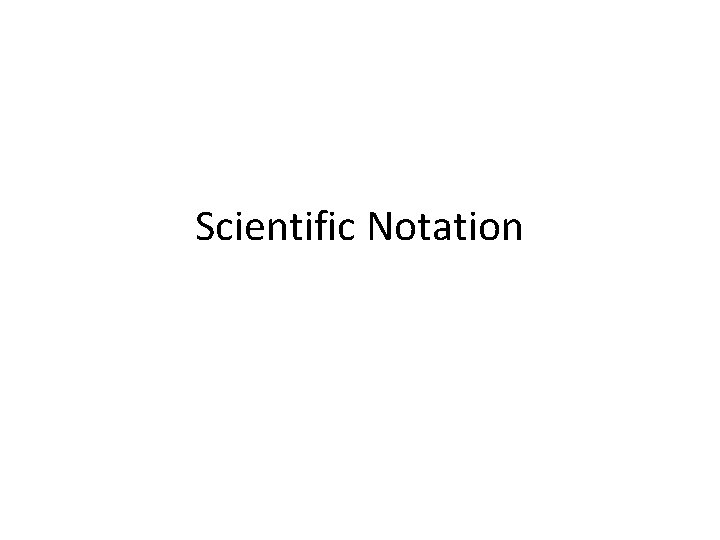Scientific Notation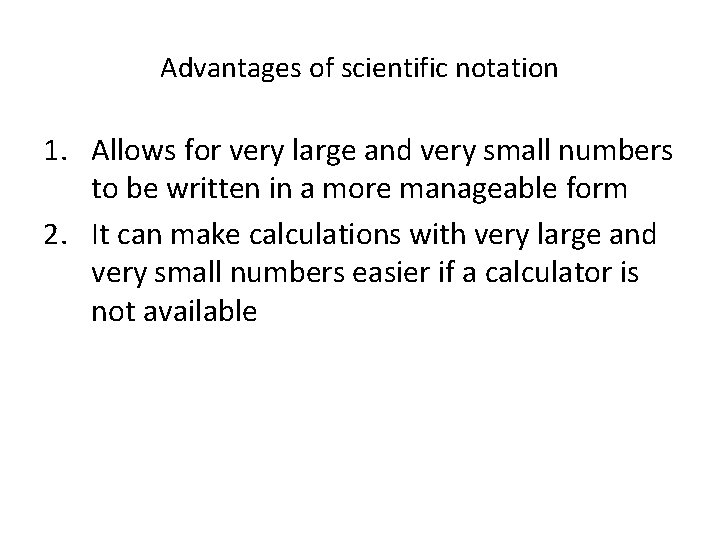Advantages of scientific notation 1. Allows for very large and very small numbers to be written in a more manageable form 2. It can make calculations with very large and very small numbers easier if a calculator is not availableThe general format of scientific notation is N x 10 y Where 1< N < 10 And y indicates the number of places and direction the decimal movesPositive exponents To convert 1234000. 000 into scientific notation we move the decimal behind the first number. Count the number of places moved and that number becomes the exponent on the 10. 1234000. 000 And you get 1. 234000000 x 106To put the number back into regular notation you move the decimal back to the right the number of places stated by the exponents The exponent is 4, therefore the decimal moves 4 places. 3. 678 x 104 And you get 36780Negative Exponents • To convert 0. 00123 into scientific The number of spaces moved notation, wethe move the decimal behind becomes exponent on the digit. 10, first butnon-zero as a negative. 0. 00123 1. 23 x 10 -3Scientific Notation 65, 000 kg 6. 5 × 104 kg • Converting into Sci. Notation: – Move decimal until there’s 1 digit to its left. Places moved = exponent. – Large # (>1) positive exponent Small # (<1) negative exponent – Only include sig figs.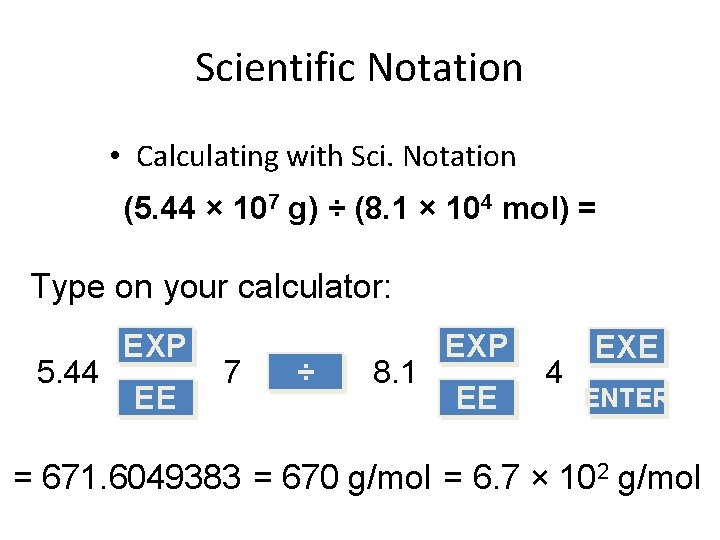Scientific Notation • Calculating with Sci. Notation (5. 44 × 107 g) ÷ (8. 1 × 104 mol) = Type on your calculator: 5. 44 EXP EE 7 ÷ 8. 1 EXP EE 4 EXE ENTER = 671. 6049383 = 670 g/mol = 6. 7 × 102 g/mol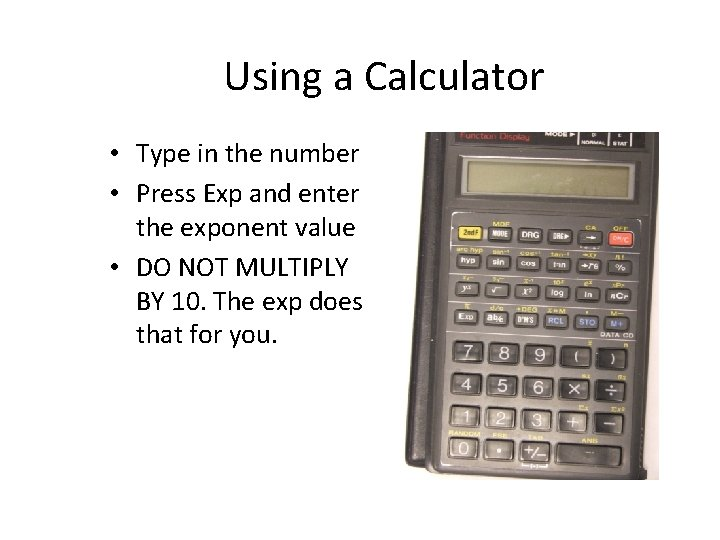Using a Calculator • Type in the number • Press Exp and enter the exponent value • DO NOT MULTIPLY BY 10. The exp does that for you.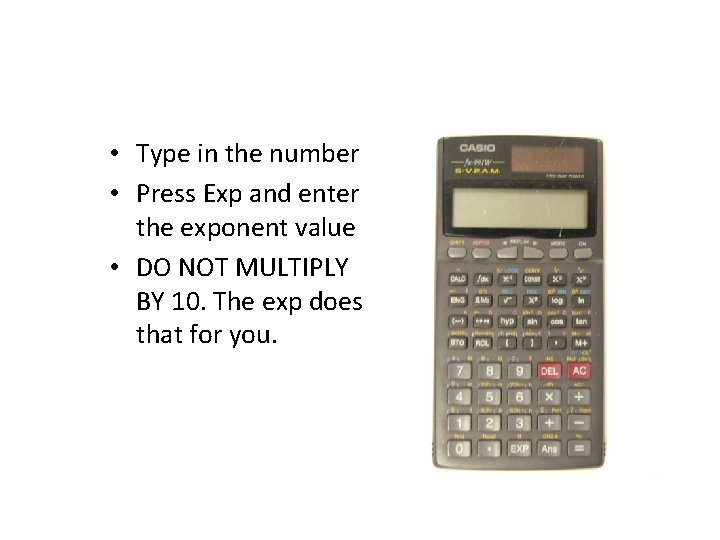• Type in the number • Press Exp and enter the exponent value • DO NOT MULTIPLY BY 10. The exp does that for you.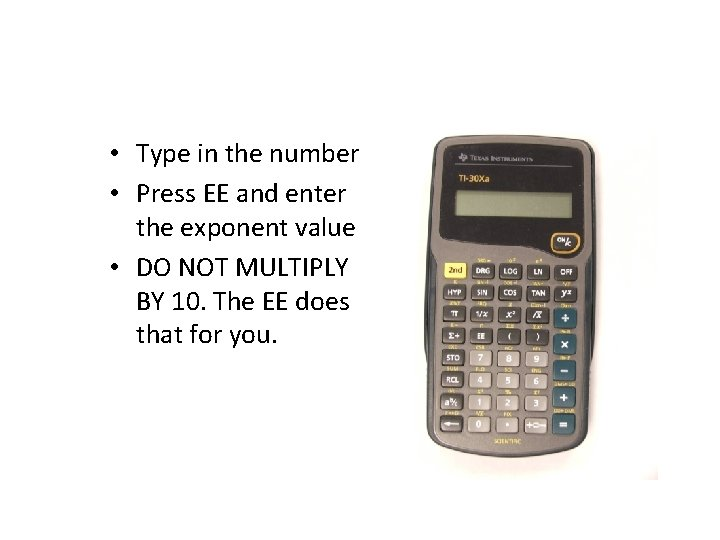• Type in the number • Press EE and enter the exponent value • DO NOT MULTIPLY BY 10. The EE does that for you.• Type in the number • Press 2 nd x-1 and enter the exponent value • DO NOT MULTIPLY BY 10. The 2 nd x-1 does that for you.• Type in the number • Press Exp and enter the exponent value • DO NOT MULTIPLY BY 10. The exp does that for you.Copying Answers from a calculator If answer on calculator looks like this X 2 E 7 X 27 Do not write it like that!!! Instead, write it as 2 x 107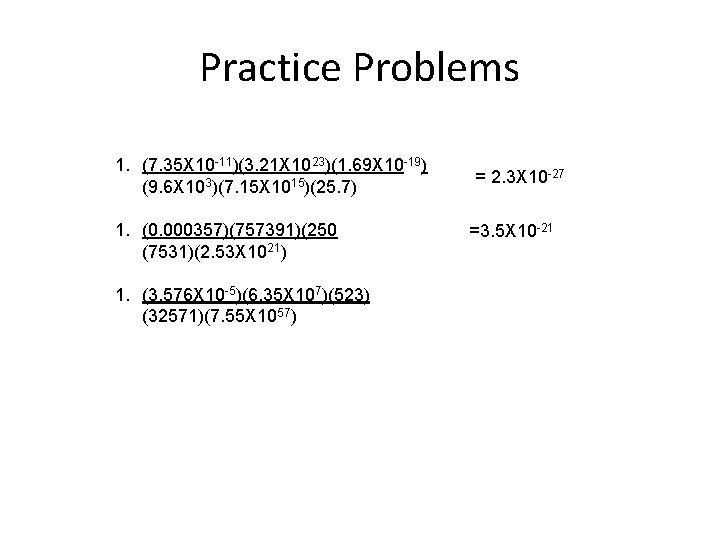Practice Problems 1. (7. 35 X 10 -11)(3. 21 X 1023)(1. 69 X 10 -19) (9. 6 X 103)(7. 15 X 1015)(25. 7) 1. (0. 000357)(757391)(250 (7531)(2. 53 X 1021) 1. (3. 576 X 10 -5)(6. 35 X 107)(523) (32571)(7. 55 X 1057) = 2. 3 X 10 -27 =3. 5 X 10 -21